Courses

# Engineering Mechanics - 2

## 20 Questions MCQ Test Mock Test Series for SSC JE Mechanical Engineering | Engineering Mechanics - 2

Description
This mock test of Engineering Mechanics - 2 for SSC helps you for every SSC entrance exam. This contains 20 Multiple Choice Questions for SSC Engineering Mechanics - 2 (mcq) to study with solutions a complete question bank. The solved questions answers in this Engineering Mechanics - 2 quiz give you a good mix of easy questions and tough questions. SSC students definitely take this Engineering Mechanics - 2 exercise for a better result in the exam. You can find other Engineering Mechanics - 2 extra questions, long questions & short questions for SSC on EduRev as well by searching above.
QUESTION: 1

### On a ladder resting on a smooth ground and leaning against vertical wall, the force of friction will be:

Solution:

Free body diagram of a ladder resting on a frictional surface is: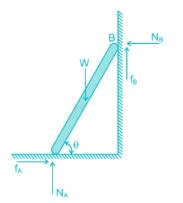The force of friction will be upwards at its upper end and towards the wall at its lower end.

As the ground is smooth (but the wall does not), so fA = 0 and fB ≠ 0

So the force of friction will be upwards at its upper end.

QUESTION: 2

### A body moves with a speed of 10 m/s in the curved path of 25 m radius of curvature. If the tangential acceleration is 3 m/s2, then total acceleration for the body will be:

Solution:

Normal component: an = v2/r = (10)2/(25) = 4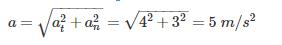QUESTION: 3

### A person who weighs 800 N steps onto a scale that is on the floor of an elevator car. If the elevator accelerates upward at a rate of 5 m/s2, what will the scale read?

Solution:

The person exerts a downward force on the scale, and the scale pushes up on the person with an equal (but opposite) force, FN. Thus, the scale reading is FN, the magnitude of the normal force.

FN – FW = ma ⇒ FN – mg = ma

⇒ F= ma + mg = m (a + g) = (W/g) (a + g) = (800/10) (10 + 5)

F= 1200 N

QUESTION: 4

Two forces of 30 N and 40 N are acting at 90° to each other, the resultant force is

Solution:

Resultant between two forces P and Q, acting at an angle θ is defined as: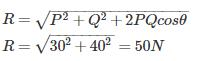QUESTION: 5

A fan consumes 20 W of electric power and discharges air from a ventilated room at 0.25 kg/s. The maximum air outlet velocity is nearly

Solution:

A fan is claimed to increase the velocity of air to a specified value while consuming electric power at a specified rate. The electric power input will be equal to the rate of increase of the kinetic energy of air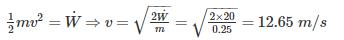QUESTION: 6

Which of the following forces have zero value of resultant?

Solution:

Equilibrium is the status of the body when it is subjected to a system of forces. If the resultant force is equal to zero it implies that the net effect of the system of forces is zero this represents the state of equilibrium.

QUESTION: 7

A man stands on weighing scale in a lift which carries him upwards with acceleration. The reading on the weighing scale will be

Solution:

Using Newton’s Second law of motion:

R−mg=ma⇒R=m(g+a)R−mg=ma⇒R=m(g+a)

So apparent weight will be greater than the true weight.

QUESTION: 8

An elevator weighing 1000 kg attains an upward velocity of 4 m/sec in two seconds with uniform acceleration. The tension in the supporting cables will be:-

Solution: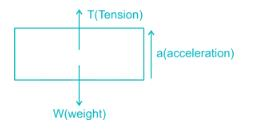Force = T - W

ma = T - W

Acceleration is given as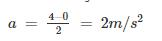Tension is given as

T = mg + ma = m (g + a)

T = 1000 (10 + 2)

T = 12000 N

QUESTION: 9

A barge is pulled by two tugboats as shown in the figure below. The resultant of forces exerted by the tugboats is 1000 kg force. What will be the value of θ so that tension in rope 2 is minimum?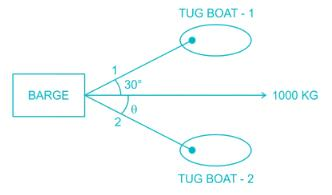Solution: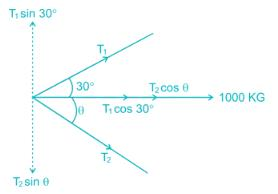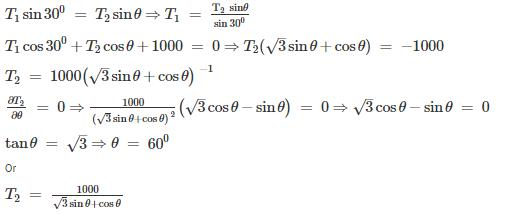By taking the values of θ, given in the options we can conclude that for θ = 600, T2 is minimum.

QUESTION: 10

The Force applied on a body of mass 100 kg to produce an acceleration of 5 m/s2, is

Solution:

Acccording to Newton's Second Law of Motion:

F=ma=100×5=500kg−m/s2

QUESTION: 11

A rubber ball is dropped from a height of 2 m. If there is not loss of velocity after rebounding the ball will rise to a height of

Solution:

Conservation of energy:

P.E1+K.E1=P.E2+K.E2P.E1+K.E1=P.E2+K.E2

As there is no loss of velocity so

K.E=12mv2⇒K.E1=K.E2K.E=12mv2⇒K.E1=K.E2

P.E1=P.E1⇒h1=h2⇒h2=2m

QUESTION: 12

A 50 - kg box rests on horizontal floor for which coefficient of friction is 0.3. If the box is subjected to a horizontal towing force of 400 N, what is its velocity after 5 sec from rest? (Take g = 10 m/s2

Solution: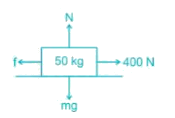Initially checking whether towing force can overcome the static friction force or not.

Friction force (f) = μN = μmg = 0.3 × 50 × 10 = 150 N

∴ Net force on box = 400 – 150 = 250 N

⇒ 250 = ma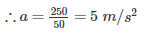Velocity after 5 second

V = u + at = 0 + 5 × 5 = 25 m/s

QUESTION: 13

A cannonball is fired from a tower 80 m above the ground with a horizontal velocity of 100 m/s. Determine the horizontal distance at which the ball will hit the ground. (Take g = 10 m/s2)

Solution: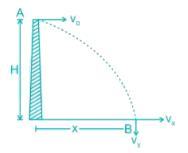vx = vo = Horizontal component of velocity

vy = Vertical component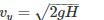Time taken by the body to cover vertical distance H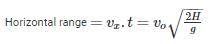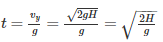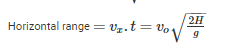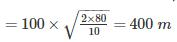QUESTION: 14

A bucket of water weighing 10 kg is pulled up from a 20 m deep well by a rope weighing 1 kg/m length, then the work done is _____.

Solution:

Let at a distance of x, weight to be pulled is 10 kg of bucket and x kg of rope weight.

So total weight is (10+x)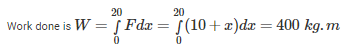QUESTION: 15

The sum of kinetic and potential energy of a falling body _____.

Solution:

Kinetic energy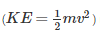represents energy the mass possesses by virtue of its motion. Likewise, potential energy (PE=mgh) represents energy the mass possesses by virtue of its position.

E=KE+PE

E is the total energy of the mass: i.e., the sum of its kinetic and potential energies. It is clear that E is a conserved quantity: i.e., although the kinetic and potential energies of the mass vary as it falls, its total energy remains the same and one form of energy is being converted into other form of energy.

QUESTION: 16

In case of principal axes of a section _____.

Solution:

In principal axes of a section product of inertia is zero.

Product of inertia:- The moment of inertia between any two mutually perpendicular axis in the plane of area is the produce of inertia.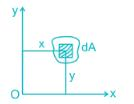Product of inertia,

Ixy=∫dA.xy

QUESTION: 17

If v1 and v2 are the initial velocities of two bodies making direct collision and if u1 and u2 are their respective velocities after collision then the coefficient of restitution is given by:

Solution:

The energy dissipation during impact is called by the term, coefficient of restitution, a scalar quantity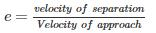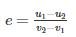QUESTION: 18

The position of a particle in rectilinear motion is given by the equation (x = t3 - 2t2 + 10t - 4), where x is in meters and t is in seconds. What will be the velocity of the particle at 3s?

Solution:

Position (x) = t3 – 2t2 + 10t – 4

Velocity (v) =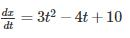At t = 3 s

V = 3 × 32 – 4 × 3 + 10 = 25 m/s

QUESTION: 19

The angular velocity (in radians /sec) of a body rotating at N rpm is:

Solution:

Rpm is just a measure of how many times a point is revolving per minute.

In a single revolution, a point rotates an angle = 2π

So, the total angle a point is rotating per minute = N x 2 π

The angular velocity is a measure of how fast it is rotating = Angle Rotated/Time Required = 2π N/60

QUESTION: 20

For maximum horizontal range, the angle of projection of a projectile should be

Solution:

Horizontal range,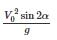For maximum horizontal range.

Sin 2α = 1

Or 2α = 90°

Or α = 45°